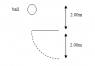# Centripetal force problems for dynamics

• pinkyboo
Centripetal force would be the force pushing the car in a straight line. Frictional force would be the force stopping the car from moving.

#### pinkyboo

Centripetal force problems for dynamics :)

## Homework Statement

I have two questions!

1. You are driving at a speed of 60.0km/h around a turn with a radius of 75m. The coefficient of friction between the tires and the road is 0.85.
a. After traveling 20° around the turn, you hit a patch of ice and your coefficient of friction is reduced to 0.15. After 5 seconds, where are you with respect to the start of the turn?
b. Repeat question a. with a banked curve of 35°.

2. A 2.00kg ball is dropped, it sticks to a rope, and makes a circular motion (see below). What is the net force on the banana at the bottom of the swing. (Assume the velocity is constant once it hits the rope... or, for more accuracy, use the conservation of energy formulas)

Fnet = mac

ac = mv2/r

ac = 4∏2r/T2

## The Attempt at a Solution

(first question)

v = 16.6666667m/s, r = 75m, μ = 0.85

For this one, I'm not even really sure where to start. :(

I wanted to do the Fnet = m x 4∏2r/T2 equation, but since I don't have the period, I wasn't really sure where to go at all. Furthermore, part b just boggles meI also wanted to find FN or just do something concerning the normal or friction forces since I do have the coefficient of friction, but again, without the mass, I'm at a loss.

(second question)

I attached the diagram of the question.

m = 2.00 kg

Grrrr... with this one, I tried to make an FBD, but all attempts were futile! I tried to make Fgx in the direction of the motion, but I'm at a loss for this question as well.

Sorry my attempts at any solutions seem so juvenile, but I really am not sure what to do. Any help would be greatly appreciated! :rofl:

#### Attachments

•question 3 ahh.PNG
1.4 KB · Views: 372
First calculate the angular velocity ω = v/r. Then the centripetal force is Fc = m ω2 r. The frictional force is Ff = μ m g. So we have eqilibrium if m ω2 r < μ m g. g is the gravitational acceleration downwards and r and v are the radius and velocity. Notice that m cancels out.

Can you take it from here ?

Last edited:
Thank you! I get now how you canceled the masses, but as for 'ω'... I just searched it, and though I do understand it, it's not something we learned in my school. :( would I be able to use Fc = m x 4∏2r/T2?

And after this step, I'm at 451.828058 m/s with the velocity...

and do you have any idea for how I would go about solving parts a and b? I'm really bad at this. :( sorry for all the questions!

Angular velocity is the rate an angle(in radians) is changing. It is 1/(2 π T).

Problem a)

1. Find the centripetal force.
2. Find the frictional force for μ=0.85
3. Same as 2 for μ=0.15
4. get 1-3. This is the force now making the car slide

Think about it. Draw the car at the point it starts to slide and resolve the forces.